Refer to our Texas Go Math Grade 8 Answer Key Pdf to score good marks in the exams. Test yourself by practicing the problems from Texas Go Math Grade 8 Module 5 Quiz Answer Key.

5.1 Writing Linear Equations from Situations and Graphs

Write the equation of each line in slope-intercept form.

Question 1.The standard form of an equation for the linear function is y = mx + b. To write an equation in slope-intercept form, we need to get values for the slope and y-intercept.

We can read the starting point from the graph, which is the intersection on the y-axis when x = 0. So we can read what our value is for the y-intercept. → starting point is (0, b)
Thus, b = 20.

The slope is calculated by:
m = $$\frac{y_{2}-y_{1}}{x_{2}-x_{1}}$$
Choose two points from the graph and put them in thecalculation I’ll take (0, 20) for (x1, y1) and (2, 80) for (x2, y2)
m = $$\frac{80-20}{2-0}$$
m = $$\frac{60}{2}$$
m = 30
The equation in slope-intercept form is: y = 30x + 20

Module 5 Test Answers Texas Go Math Grade 8 Pdf Question 2.The standard form of an equation for the linear function is y = mx + b. To write an equation in slope-intercept form, we need to get values for the slope and y-intercept.

We can read the starting point from the graph, which is the intersection on the y-axis when x = 0. So we can read what our value is for the y-intercept. → starting point is (0, b)
Thus, b = 60

The slope is calculated by:
$$\frac{y_{2}-y_{1}}{x_{2}-x_{1}}$$

Choose two points from the graph and put them in the calculation (0, 60) for (x1, y1) and (6, 0) for (x2, y2)
m = $$\frac{0-60}{6-0}$$
m = $$\frac{-60}{6}$$
m = -10
The equation in slope-intercept form is: y = -10x + 60

5.2 Writing Linear Equations from a Table

Write the equation of each linear relationship in slope-intercept form.

Question 3.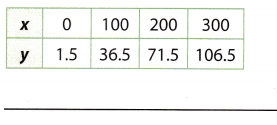The standard form of an equation for the linear function is y = mx + b. To write an equation in slope-intercept form, we need to get values for the slope and y-intercept.

We can read the starting point from the graph, which is the intersection on the y-axis when x = 0. So we can read what our value is for the y-intercept. → starting point is (0, b)
Thus, b = 1.5

The slope is calculated by:
$$\frac{y_{2}-y_{1}}{x_{2}-x_{1}}$$

Choose two points from the graph and put them in the calculation (0, 1.5) for (x1, y1) and (100, 36.5) for (x2, y2)
m = $$\frac{36.5-1.5}{100-0}$$
m = $$\frac{35}{100}$$
m = 0.35
The equation in slope-intercept form is: y = 0.35x + 1.5The standard form of an equation for the linear function is y = mx + b. To write an equation in slope-intercept form, we need to get values for the slope and y-intercept.

The slope is calculated by:
$$\frac{y_{2}-y_{1}}{x_{2}-x_{1}}$$

Choose two points from the graph and put them in the calculation (25, 94) for (x1, y1) and (35, 88) for (x2, y2)
m = $$\frac{88-94}{35-25}$$
m = $$\frac{-6}{10}$$
m = -0.6
To find the y-intercept (b), we can include one point from the table and the value of the slope in the standard form of the equation. So:
y = mx + b
94 = -0.6 . 25 + b
94 = -15 + b
b = 94 + 15
b = 109

The equation in slope-intercept form is: y = 0.6x + 109

5.3 Linear Relationships and Bivariate Data

Write the equation of the line that connects each set of data points.

Question 5.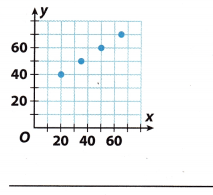The standard form of an equation for the linear function is y = mx + b. To write an equation in slope-intercept form, we need to get values for the slope and y-intercept.

The slope is calculated by:
$$\frac{y_{2}-y_{1}}{x_{2}-x_{1}}$$

Choose two points from the graph and put them in the calculation (20, 40) for (x1, y1) and (50, 60) for (x2, y2)
m = $$\frac{60-40}{50-20}$$
m = $$\frac{20}{30}$$
m = $$\frac{2}{3}$$
m ≈ 0.67

To find the y-intercept (b), we can include one point from the table and the value of the slope in the standard form of the equation. So:
y = mx + b
40 = 0.67 . 20 + b
40 = 13.4 + b
b = 40 – 13.4
b ≈ 26.6
The equation in slope-intercept form is: y = 0.67x + 26.6

Question 6.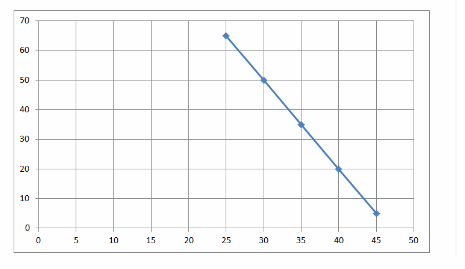Confirm the points represent a linear relationship

Slope = $$\frac{50-65}{30-25}$$ = $$\frac{-15}{5}$$ = -3
Find the slope using given points from the table by Slope(m) = $$\frac{y_{2}-y_{1}}{x_{2}-x_{1}}$$ where
(x2, y2) = (30, 50) and (x1, y1) = (25, 65)
50 = -3(30) + b Substituting the value of slope (m) and (x, y) in the slope-intercept form to find y-intercept (b):
50 = -90 + b y = mx + b
b = 50 + 90 = 140
y = -3x + 140 Substitute the value of slope (m) and y-intercept (b) in slope-intercept form: y = mx + b

Essential Question

Go Math 8th Grade Pdf Module 5 Linear Functions Answer Key Question 7.
Write a real-world situation that can be represented by a linear relationship.
A linear equation uses one or more variables where one variable is independent of the other. The degree of the linear equation is one.
Imagine that you are taking a cab while on vacation. You know that the cab service charges $10 to pick your family up from your hotel and another$0.20 per mile for the trip. Without knowing how many miles it will be to each destination, you can set up a linear equation that can be used to find the cost of any taxi trip you take on your trip. By using “x” to represent the number of miles to your destination and “y” to represent the cost of that taxi ride, the linear equation would be y = 0.20x + 10.

Texas Go Math Grade 8 Module 5 Mixed Review Texas Test Prep Answer Key

Selected Response

Question 1.
An hourglass is turned over with the top part filled with sand. After 3 minutes, there are 855 mm of sand in the top half. After 10 minutes, there are 750 mm of sand in the top half. Which equation represents this situation?
(A) y = 285x
(B) y = -10.5x + 900
(C) y = -15x + 900
(D) y = 75x
(C) y = -15x + 900

Explanation:
(3, 855) Write the given information as ordered pair

(10, 750)

Slope = $$\frac{855-750}{3-10}$$ = $$\frac{105}{-7}$$ = -15 Find the slope using given points by Slope (m) = $$\frac{y_{2}-y_{1}}{x_{2}-x_{1}}$$

750 = -15(10) + b Substituting the value of slope (m) and (x, y) in the
750 = -15(10) + b slope intercept form to find y-intercept (b):
750= -150 + b
b = 750 + 150 = 900y = mx + b
y = -15x + 900 Substitute the value of slope (m) and y-intercept (b) in slope-intercept form:
y = mx + b

Question 2.
Which graph shows a linear relationship?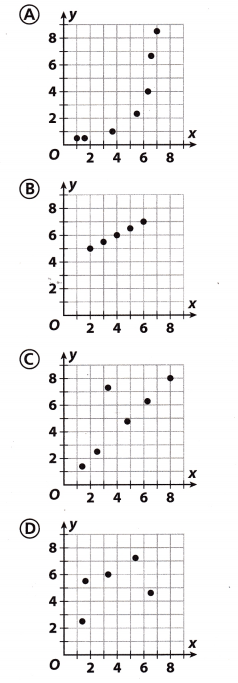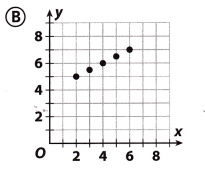Graph B represents the linear relationship The data appears to lie on a straight line

8th Grade Math Module 5 Writing Linear Equations Module Quiz Answer Key Question 3.
What are the slope and y-intercept of the relationship shown in the table?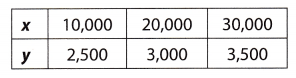(A) slope = 0.05, y-intercept = 1,500
(B) slope = 0.5, y-intercept = 1,500
(C) slope = 0.05, y-intercept = 2,000
(D) slope = 0.5, y-intercept = 2,000
(C) slope = 0.05, y-intercept = 2,000

Explanation:
slope = $$\frac{3000-2500}{20000-10000}$$ = $$\frac{500}{10000}$$ = 0.05
Find the slope using given points from the table by Slope(m) = $$\frac{y_{2}-y_{1}}{x_{2}-x_{1}}$$ where (x2, y2) = (20000, 3000) and (x1, y1) = (10000, 2500)

3000 = 0.05(2000) + b Substituting the value of slope (m) and (x, y) in the slope-intercept form to find y-intercept (b):
3000 = 1000 + b y = mx + b
b = 3000 – 1000 = 2000

Gridded Response

Question 4.
Franklin’s faucet was leaking water, so he put a bucket underneath to catch the water. After a while, Franklin started keeping track of how much water was leaking. His data is in the table below.Predict how many gallons of water will have leaked if Franklin hasn’t stopped the leak after 14 hours.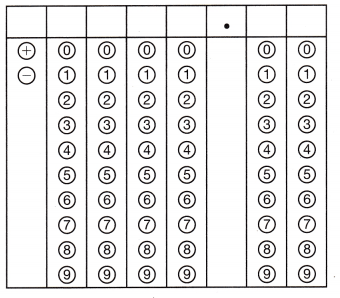10